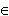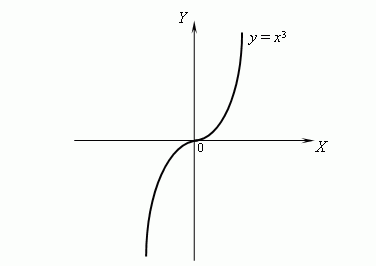# Convexity, concavity and inflexion points of a function

The second derivative. Convex and concave function.
Sufficient condition of concavity ( convexity ) of a function.
Inflexion point.

The second derivative. If a derivative f ' ( x ) of a function f ( x ) is differentiable in the point ( x 0 ), then its derivative is called the second derivative of the function f ( x ) in the point ( x 0 ) and denoted as f '' ( x 0 ).
A function f ( x ) is called convex in an interval ( a , b ), if the function f ( x ) graph is placed in this interval lower than a tangent line, going through any point ( x 0 , f ( x 0 ) ), x 0( a , b ).
A function f ( x ) is called concave in an interval ( a , b ), if the function f ( x ) graph is placed in this interval higher than a tangent line, going through any point ( x 0 , f ( x 0 ) ), x 0( a , b ).

Sufficient condition of concavity (convexity) of a function.
Let a function f ( x ) be twice differentiable (i.e. it has the second derivative) in an interval ( a , b ), then: if f '' ( x ) > 0 for any x( a , b ), then the function f ( x ) is concave in the interval ( a , b ); if f '' ( x ) < 0 for any x( a , b ), then the function f ( x ) is convex in the interval ( a , b ).

If a function changes a convexity to a concavity or vice versa at passage through some point, then this point is called an inflexion point an inflexion point. Hence it follows, that if the second derivative f '' exists in an inflexion point x 0 , then f '' ( x 0 ) = 0.

 E x a m p l e. Consider a graph of the function y = x 3 :This function is concave at x > 0 and convex at x < 0. In fact, y'' = 6 x , but 6 x > 0 at x < 0 and 6 x < 0 at x < 0, hence, y'' > 0 at x 3 0 and y'' < 0 at x < 0, hence it follows, that the function y = x 3 is concave at x > 0 and convex at x < 0. Then the point x = 0 is the inflexion point of the function y = x 3 .